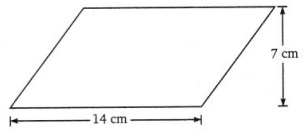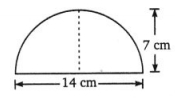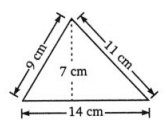### Grade 8 Maths Mensuration Multiple Choice Questions (MCQs)

1. The area of triangle is:
(a) base × height
(b)$\frac{1}{2}$ × base × height
(c)$\frac{1}{2}$ × (base + height)
(d) base + height

2. ∆ ABC is isosceles is which AE ⊥ BC, AE = 6 cm, BC = 9 cm, the area of ∆ ABC is:
(a) 27 cm2
(b) 54 cm2
(c) 22.5 cm2
(d) 45 cm2

3. The area of parallelogram is:
(a) base + height
(b) base × height
(c) base × base
(d) height × height

4. The base in the area of parallelogram is:
(a)$\frac{\text { area }}{\text { height }}$
(b)$\frac{\text { height }}{\text { area }}$
(c) base × base
(d) area × height

5. The area of the given alongside parallelogram:
(a) 98 cm2
(b) 77 cm2
(c) 49 cm2
(d) None of these6. The area of the given alongside rectangle:
(a) 98 cm2
(b) 77 cm2
(c) 49 cm2
(d) None of these7. The circumference of circle whose diameter is 14 cm will be:
(a) 44 cm
(b) 88 cm
(c) 44 cm2
(d) 88 cm2

8. The perimeter of circle is its ……………. .
(a) area
(b) circumference
(d) diameter

9. Diameter is ……………… .

10. π (pi) is:
(a) ratio of circumference to diameter
(b) diameter of circumference
(c)$\frac{21}{17}$
(d) 3.41

11. The area of the given alongside figure:
(a) 98 cm2
(b) 77 cm2
(c) 49 cm2
(d) None of these12. The area of the given alongside triangle:
(a) 98 cm2
(b) 77 cm2
(c) 49 cm2
(d) None of these13. What will be the area of circular button of radius 7 cm?
(a) 154 cm2
(b) 49 cm2
(c) 154 cm
(d) 3.14 × 7 cm2

14. 1 m2 = ……………… .
(a) 100 cm2
(b) 1000 cm2
(c) 10000 m2
(d) 10000 cm2

15. One hectare is equal to:
(a) 100 cm2
(b) 1000 cm2
(c) 10000 m2
(d) 10000 cm2

16. The perimeter of circular field is 242 cm. The area of the field is:
(a) 9317 cm2
(b) 18634 cm2
(c) 4658.5 cm2
(d) None of these

17. The difference between the circumference and radius of a circle is 37 cm. The area of the circle is:
(a) 111cm2
(b) 184 cm2
(c) 154 cm2
(d) 259 cm2

18. The circumference of two circles are in the ratio 2:3. The ratio of their areas is:
(a) 2:3
(b) 4 : 9
(c) 9 : 4
(d) None of these

19. On increasing the diameter of circle by 40%, its area will be increased by:
(a) 40%
(b) 80%
(c) 96%
(d) None of these

20. The surface area of a cuboid is:
(a) 2(lb + bh + lh)
(b) 3(lb + bh + lh)
(c) 2(lb – bh – lh)
(d) 3(lb – bh – lh)

### Class 8 Maths Mensuration Fill In The Blanks

1. The area of a rhombus whose diagonals are of length 8 cm and 5 cm is …………….. .
2. Curved surface area of a cylinder having radius ‘r’ and height ‘h’ is ……………… .
3. The side of a cube whose surface area is 294 cm2, is ……………….. .
4. The capacity of a cube of edge 10 cm is ……………… .
5. 10000 cm3 = ……………… liters.

### Class 8 Maths Mensuration True(T) Or False(F)

1. Every rectangle is a square.
2. Diagonals of a rhombus bisect at right angles.
3. Every rectangle is a parallelogram.
4. Both diagonals of a parallelogram are equal.
5. Rhombus is a parallelogram with all sides equal.

### Class 8 Maths Mensuration Very Short Answer Type Questions

1. Find the area and perimeter of the rectangle having length = 15 cm and breadth = 11 cm.
2. Find the area of a parallelogram with base 5.2 cm and height 2 cm.
3. The area of a triangle of height 13 m is 507 m2. Find its base.
4. The edge of a cube is 24 cm. How many cubes of 8 cm side can be formed from this cube?
5. Find the volume of the cylinder whose base diameter is 14 cm and height is 10 cm.
6. The diameter of a garden roller is 2 m and it is 3 m long. How much area will it cover 5 revolutions?

### Class 8 Maths Mensuration Short Answer Type Questions

1. Area of a rhombus is 24 cm2. If one of its diagonal is 6 cm then its perimeter is ………… cm.
2. A cylindrical tower is 5 m in diameter and 14 m. Find the cost of painting its curved surface at ₹ 25 per m2.
3. The internal dimensions of a hall are in the ratio 7 : 5 : 3. If the volume of the room is 2835 m2, then find then length of the hall.
4. Wheel of a cycle with diameter 56 cm completes 100 revolutions to reach the destination. Find the total distance covered.

### Class 8 Maths Mensuration Long Answer Type Questions

1. What is the perimeter of the figure.2. Two cylinders have the same radii of bases if their heights are 15 cm and 25 cm, then find the ratio of their volumes.
3. The ratio of radii of two spheres is 1 : 3
(a) What is the ratio of their volumes?
(b) What is ther ratio of their surface areas?